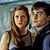# Only 20% of Adults Over 30 Can Pass This Calculus Test

## Calculus is one of the hardest form of math! Only some adults can pass this super difficult test, can you?Created by Carly Wallace
On Feb 4, 2023

Not everyone can pass this super challenging calculus test. Calculus is the mathematical study of continuous change and is often used in structural engineering or architecture. We've put together some of the hardest calculus questions together. So take out your calculator and try your hand at this math quiz!

1 / 10

What is the derivative of (x + 1) sin x?

2 / 10

If x = 2 ln cot t and y = tan t + cot t, the value of dy/dx is:

3 / 10

D[f(x). g(x). h(x)] = {g(x). h(x)} * D[f(x)] + {f(x). h(x)} * D[g(x)] + {f(x). g(x)} * D[h(x)] is the:

4 / 10

Integral of the constant function f(x) = k is:

5 / 10

What is the integral of the function f(x) = sin 2x?

6 / 10

Determine all the value of c, for the function
f(x) = x^3 +6
for the interval [1, 3] which satisfies the mean value theorem (approx).

7 / 10

For a function f(x) to satisfy the mean value theorem over (a, b), the function should be continuous on:

8 / 10

Determine all the value of c, for the function
f(x) = 4x^2
for the interval [2, 5] which satisfies the mean value theorem (approx).

9 / 10

Evaluate the approximate value of the definite integral of ln(x) over [2,4]

10 / 10

The quotient rule is used to find the:

10
Questions left

### Which math subject were you best at in school?

Calculating results
These are 10 of the World CRAZIEST Ice Cream Flavors
Created by Tal Garner
On Nov 18, 2021